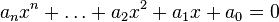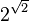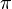# Transcendental number facts for kids

Kids Encyclopedia Facts

A real or complex number is called a transcendental number if it cannot be found as a result of an algebraic equation with integer coefficients.$a_{n}x^{n} + \dots + a_{2}x^2 + a_{1}x + a_{0} = 0$

Proving that a certain number is transcendental can be very hard. Each transcendental number is also an irrational number. The first people to see that there were transcendental numbers were Gottfried Wilhelm Leibniz and Leonhard Euler. The first to actually prove there were transcendental numbers was Joseph Liouville. He did this in 1844.

Well known transcendental numbers:

• e
• π
• ea for algebraic a 0
•$2^{\sqrt{2}}$
• David Hilbert, "Über die Transcendenz der Zahlen$e$ und$\pi$", Mathematische Annalen 43:216–219 (1893).
• Alan Baker, Transcendental Number Theory, Cambridge University Press, 1975, .Transcendental number Facts for Kids. Kiddle Encyclopedia.